Updating search results...

# 23 Results

View
Selected filters:
• Trigonometry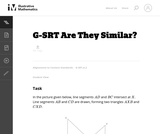Unrestricted Use
CC BY
Rating
0.0 stars

In this problem, students are given a picture of two triangles that appear to be similar, but whose similarity cannot be proven without further information. Asking students to provide a sequence of similarity transformations that maps one triangle to the other focuses them on the work of standard G-SRT.2, using the definition of similarity in terms of similarity transformations.

Subject:
Mathematics
Geometry
Trigonometry
Material Type:
Activity/Lab
Provider:
Illustrative Mathematics
Provider Set:
Illustrative Mathematics
Author:
Illustrative Mathematics
05/01/2012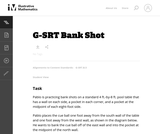Unrestricted Use
CC BY
Rating
0.0 stars

This task asks students to use similarity to solve a problem in a context that will be familiar to many, though most students are accustomed to using intuition rather than geometric reasoning to set up the shot.

Subject:
Mathematics
Geometry
Trigonometry
Material Type:
Activity/Lab
Provider:
Illustrative Mathematics
Provider Set:
Illustrative Mathematics
Author:
Illustrative Mathematics
08/21/2012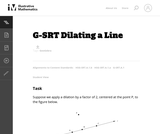Unrestricted Use
CC BY
Rating
0.0 stars

This task gives students the opportunity to verify that a dilation takes a line that does not pass through the center to a line parallel to the original line, and to verify that a dilation of a line segment (whether it passes through the center or not) is longer or shorter by the scale factor.

Subject:
Mathematics
Geometry
Trigonometry
Material Type:
Activity/Lab
Provider:
Illustrative Mathematics
Provider Set:
Illustrative Mathematics
Author:
Illustrative Mathematics
05/01/2012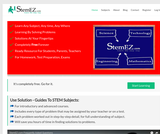Rating
0.0 stars

Encyclopedia of STEM education is a free resource with 50,000 problems/solutions covering 13 math subjects, 13 science subjects and 13 engineering subjects for self study learning, classroom teaching, tutoring and teacher training.

Subject:
Engineering
Technology
Education
Mathematics
Algebra
Calculus
Functions
Geometry
Statistics and Probability
Trigonometry
Physical Science
Chemistry
Physics
Material Type:
Full Course
Homework/Assignment
Student Guide
Teaching/Learning Strategy
Author:
open source
12/21/2017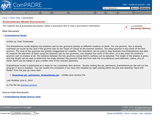Unrestricted Use
CC BY
Rating
0.0 stars

The Eratosthenes model displays the shadows cast by two gnomons (sticks) at different locations on Earth. For one gnomon, Sun is directly overhead (as would be the case if the gnomon was on the Tropic of Cancer at the summer solstice). The other gnomon is due north of the first gnomon. The sizes of the gnomons are greatly exaggerated for visibility. This simulation can be used to help illustrate how Eratosthenes was able to measure the diameter of Earth using the shadows cast by two gnomons, one situated due north of the other, on a day when the southerly gnomon cast no shadow at all. The distance between the two gnomons (along Earth's surface) can be adjusted. The length of the shadow is given, and this length can be used to determine the angle between the gnomon lines and from that the circumference (and diameter, radius, etc) of Earth. Earth can be hidden to give a better view of the relevant geometry. Eratosthenes model is distributed as a ready-to-run (compiled) Java archive. Double clicking the ejs_astronomy_Eratosthenes.jar file will run the program if Java is installed. You can modify this simulation if you have EJS installed by right-clicking within the plot and selecting "Open EJS Model" from the pop-up menu item.

Subject:
Computing and Information
Education
History, Law, Politics
Mathematics
Trigonometry
Geoscience
Physics
Space Science
Material Type:
Full Course
Interactive
Provider:
Provider Set:
ComPADRE: Resources for Physics and Astronomy Education
Author:
Todd Timberlake
01/02/2010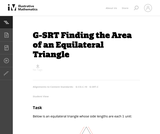Conditional Remix & Share Permitted
CC BY-NC-SA
Rating
0.0 stars

This tasks examines how to calculate the area of an equilateral triangle using high school geometry.

Subject:
Mathematics
Geometry
Trigonometry
Material Type:
Activity/Lab
Provider:
Illustrative Mathematics
Provider Set:
Illustrative Mathematics
Author:
Illustrative Mathematics
01/20/2013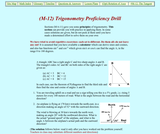Read the Fine Print
Rating
0.0 stars

This web page provides an elementary trigonometry proficiency drill. Related pages are also linked to this page. This is part of an extensive web site, "From Stargazers to Starships", that uses the topics of space exploration and space science to introduce topics in physics and astronomy.

Subject:
Education
Mathematics
Trigonometry
Physics
Material Type:
Assessment
Lecture Notes
Provider:
Provider Set:
ComPADRE: Resources for Physics and Astronomy Education
Author:
David Stern
05/14/2008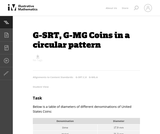Unrestricted Use
CC BY
Rating
0.0 stars

This task complements ``Seven Circles'' I, II, and III. This is a hands-on activity which students could work on at many different levels and the activity leads to many interesting questions for further investigation.

Subject:
Mathematics
Geometry
Trigonometry
Material Type:
Activity/Lab
Provider:
Illustrative Mathematics
Provider Set:
Illustrative Mathematics
Author:
Illustrative Mathematics
08/21/2012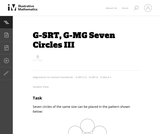Unrestricted Use
CC BY
Rating
0.0 stars

This task provides an opportunity to model a concrete situation with mathematics. Once a representative picture of the situation described in the problem is drawn (the teacher may provide guidance here as necessary), the solution of the task requires an understanding of the definition of the sine function. When the task is complete, new insight is shed on the ``Seven Circles I'' problem which initiated this investigation as is noted at the end of the solution.

Subject:
Mathematics
Geometry
Trigonometry
Material Type:
Activity/Lab
Provider:
Illustrative Mathematics
Provider Set:
Illustrative Mathematics
Author:
Illustrative Mathematics
08/21/2012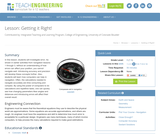Read the Fine Print
Educational Use
Rating
0.0 stars

In this lesson, students will investigate error. As shown in earlier activities from navigation lessons 1 through 3, without an understanding of how errors can affect your position, you cannot navigate well. Introducing accuracy and precision will develop these concepts further. Also, students will learn how computers can help in navigation. Often, the calculations needed to navigate accurately are time consuming and complex. By using the power of computers to do calculations and repetitive tasks, one can quickly see how changing parameters likes angles and distances and introducing errors will affect their overall result.

Subject:
Engineering
Mathematics
Geometry
Trigonometry
Geoscience
Material Type:
Activity/Lab
Lesson Plan
Provider:
TeachEngineering
Provider Set:
TeachEngineering
Author:
Janet Yowell
Jeff White
Malinda Schaefer Zarske
Matt Lippis
09/18/2014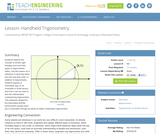Read the Fine Print
Educational Use
Rating
0.0 stars

Students explore the concept of similar right triangles and how they apply to trigonometric ratios. Use this lesson as a refresher of what trig ratios are and how they work. In addition to trigonometry, students explore a clinometer app on an Android® or iOS® device and how it can be used to test the mathematics underpinning trigonometry. This prepares student for the associated activity, during which groups each put a clinometer through its paces to better understand trigonometry.

Subject:
Engineering
Mathematics
Trigonometry
Material Type:
Lesson Plan
Provider:
TeachEngineering
Provider Set:
TeachEngineering
Author:
Scott Burns
09/18/2014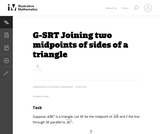Unrestricted Use
CC BY
Rating
0.0 stars

This task is closely related to very important material about similarity and ratios in geometry.

Subject:
Mathematics
Geometry
Trigonometry
Material Type:
Activity/Lab
Provider:
Illustrative Mathematics
Provider Set:
Illustrative Mathematics
Author:
Illustrative Mathematics
01/21/2013Unrestricted Use
CC BY
Rating
0.0 stars

The purpose of this task is to engage students in an open-ended modeling task that uses similarity of right triangles, and also requires the use of technology.

Subject:
Mathematics
Geometry
Trigonometry
Material Type:
Activity/Lab
Provider:
Illustrative Mathematics
Provider Set:
Illustrative Mathematics
Author:
Illustrative Mathematics
02/04/2013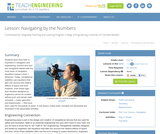Read the Fine Print
Educational Use
Rating
0.0 stars

In this lesson, students will learn that math is important in navigation and engineering. Ancient land and sea navigators started with the most basic of navigation equations (Speed x Time = Distance). Today, navigational satellites use equations that take into account the relative effects of space and time. However, even these high-tech wonders cannot be built without pure and simple math concepts basic geometry and trigonometry that have been used for thousands of years. In this lesson, these basic concepts are discussed and illustrated in the associated activities.

Subject:
Engineering
Mathematics
Geometry
Trigonometry
Geoscience
Material Type:
Activity/Lab
Lesson Plan
Provider:
TeachEngineering
Provider Set:
TeachEngineering
Author:
Janet Yowell
Jeff White
Malinda Schaefer Zarske
09/18/2014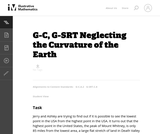Unrestricted Use
CC BY
Rating
0.0 stars

This task applies geometric concepts, namely properties of tangents to circles and of right triangles, in a modeling situation. The key geometric point in this task is to recognize that the line of sight from the mountain top towards the horizon is tangent to the earth. We can then use a right triangle where one leg is tangent to a circle and the other leg is the radius of the circle to investigate this situation.

Subject:
Mathematics
Geometry
Trigonometry
Material Type:
Activity/Lab
Provider:
Illustrative Mathematics
Provider Set:
Illustrative Mathematics
Author:
Illustrative Mathematics
03/04/2013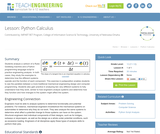Read the Fine Print
Educational Use
Rating
0.0 stars

Students analyze a cartoon of a Rube Goldberg machine and a Python programming language script to practice engineering analysis. In both cases, they study the examples to determine how the different systems operate and the function of each component. This exercise in juxtaposition enables students to see the parallels between a more traditional mechanical engineering design and computer programming. Students also gain practice in analyzing two very different systems to fully understand how they work, similar to how engineers analyze systems and determine how they function and how changes to the system might affect the system.

Subject:
Computing and Information
Engineering
Education
Mathematics
Trigonometry
Material Type:
Lesson Plan
Provider:
TeachEngineering
Provider Set:
TeachEngineering
Author:
Brian Sandall
Scott Burns
09/18/2014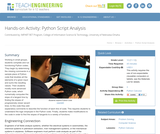Read the Fine Print
Educational Use
Rating
0.0 stars

Working in small groups, students complete and run functioning Python codes. They begin by determining the missing commands in a sample piece of Python code that doubles all the elements of a given input and sums the resulting values. Then students modify more advanced Python code, which numerically computes the slope of a tangent line by finding the slopes of progressively closer secant lines; to this code they add explanatory comments to describe the function of each line of code. This requires students to understand the logic employed in the Python code. Finally, students make modifications to the code in order to find the slopes of tangents to a variety of functions.

Subject:
Computing and Information
Engineering
Education
Mathematics
Trigonometry
Material Type:
Activity/Lab
Provider:
TeachEngineering
Provider Set:
TeachEngineering
Author:
Brian Sandall
Scott Burns
09/18/2014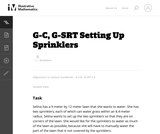Unrestricted Use
CC BY
Rating
0.0 stars

This modeling task involves several different types of geometric knowledge and problem-solving: finding areas of sectors of circles (G-C.5), using trigonometric ratios to solve right triangles (G-SRT.8), and decomposing a complicated figure involving multiple circular arcs into parts whose areas can be found (MP.7).

Subject:
Mathematics
Geometry
Trigonometry
Material Type:
Activity/Lab
Provider:
Illustrative Mathematics
Provider Set:
Illustrative Mathematics
Author:
Illustrative Mathematics
05/01/2012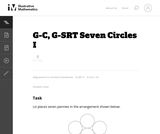Unrestricted Use
CC BY
Rating
0.0 stars

This task is intended to help model a concrete situation with geometry. Placing the seven pennies in a circular pattern is a concrete and fun experiment which leads to a genuine mathematical question: does the physical model with pennies give insight into what happens with seven circles in the plane?

Subject:
Mathematics
Geometry
Trigonometry
Material Type:
Activity/Lab
Provider:
Illustrative Mathematics
Provider Set:
Illustrative Mathematics
Author:
Illustrative Mathematics
08/20/2012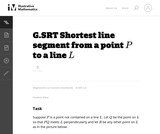Unrestricted Use
CC BY
Rating
0.0 stars

This is a foundational geometry task designed to provide a route for students to develop some fundamental geometric properties that may seem rather obvious at first glance. In this case, the fundamental property in question is that the shortest path from a point to a line meets the line at a right angle, which is crucial for many further developments in the subject.

Subject:
Mathematics
Geometry
Trigonometry
Material Type:
Activity/Lab
Provider:
Illustrative Mathematics
Provider Set:
Illustrative Mathematics
Author:
Illustrative Mathematics